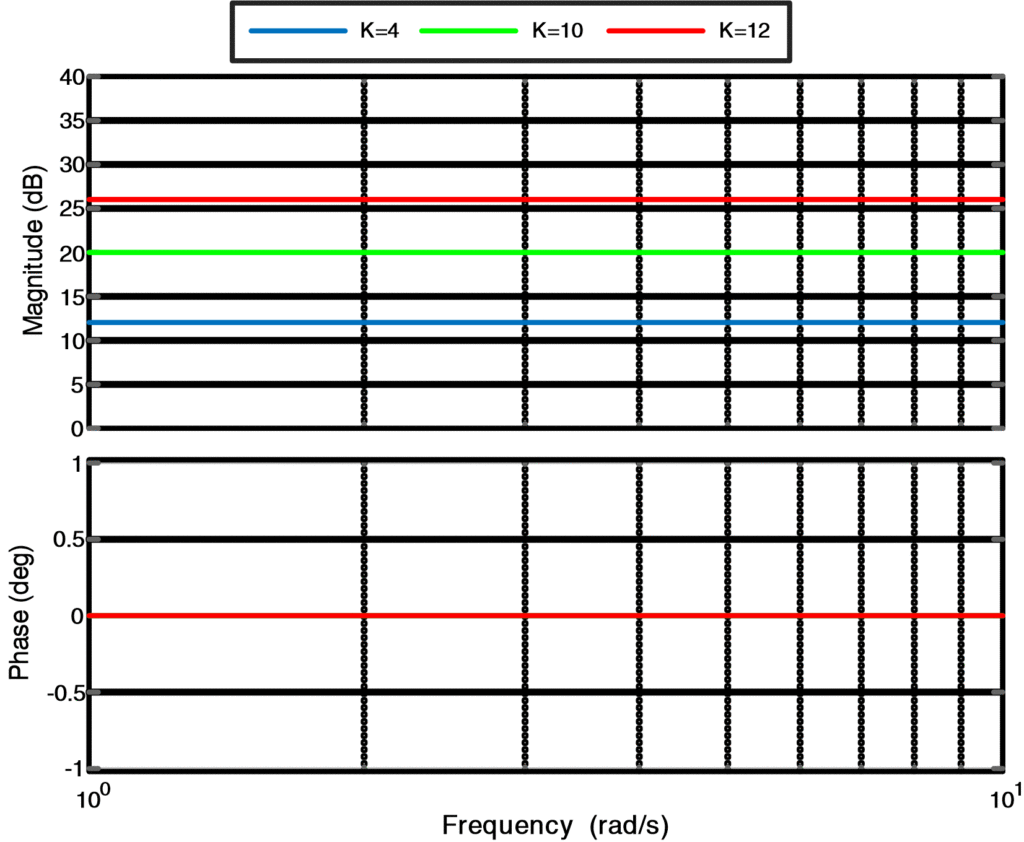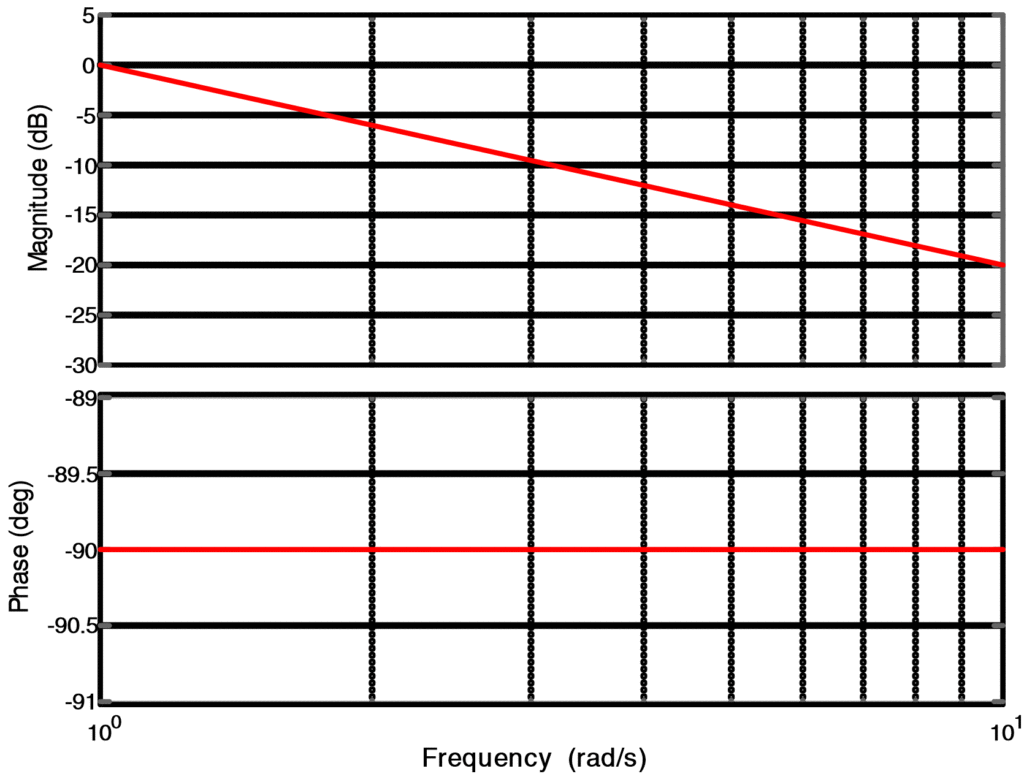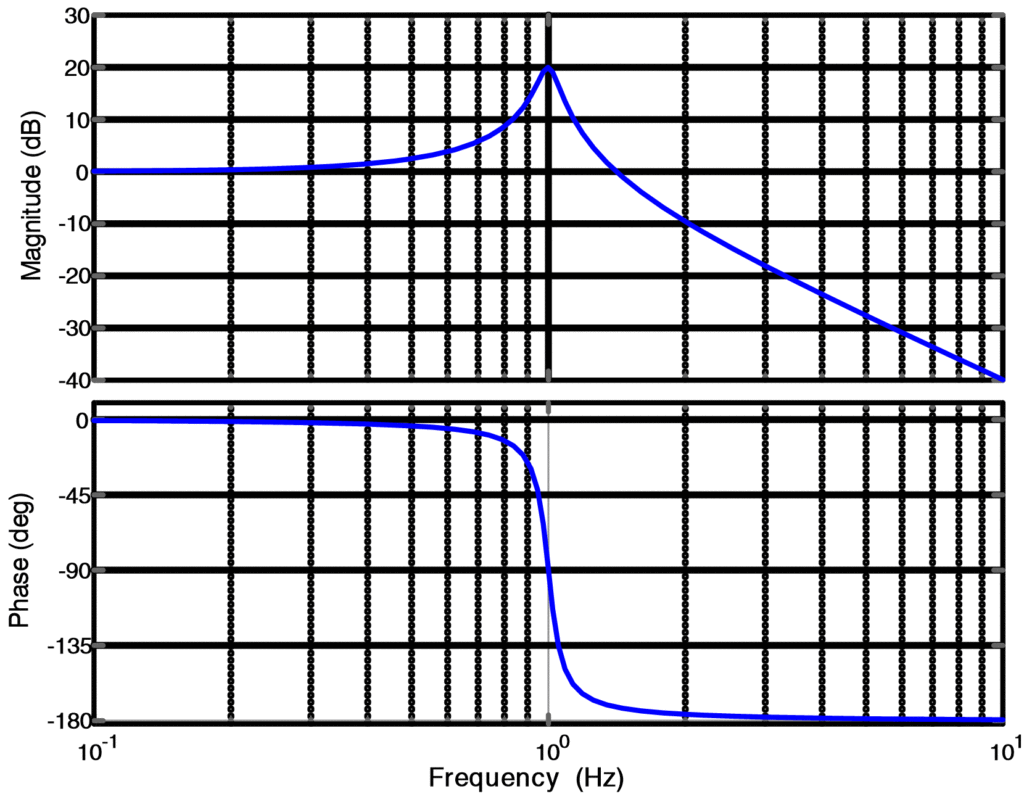Home / Control Systems / Bode Plot MATLAB | Bode Plot Transfer Function

Bode Plot MATLAB | Bode Plot Transfer Function

Want create site? Find Free WordPress Themes and plugins.

Bode Plot Definition

H.W. Bode introduced a method to present the information of a polar plot of a transfer function GH(s), actually the frequency response GH (jω), as two plots with the angular frequency were at the common axis. The first plot shows the magnitude of the transfer function as a function of ω, and the second plot shows the phase as a function of ω. This pair of plots is referred to as Bode Plot or Bode Diagram.

The magnitude of the transfer function is expressed in decibels (dB), the phase in degrees and the common parameter of frequency is plotted on a logarithmic scale in radians. At times, the magnitude of a transfer function is referred to as gain and the corresponding plot as a gain plot.

1. One apparent advantage of the bode diagram is the relative ease with which it is obtained.
2.  A second advantage is that this technique is feasible for lower frequencies, where measuring the phase difference between input and output signals is difficult.
3.  A third advantage results from the introduction of logarithms, thus reducing the process of multiplying two transfer functions to addition.

Corner frequency or Breakpoint

In bode-plot, low-frequency asymptote (that is ω<<1/T) and high-frequency asymptote (that is ω>>1/T) cut off at 0 decibels (dB) line where ω=1/T, that is the frequency called corner frequency or break point.

Bode Plot Matlab

The Bode plot or diagram of a transfer function can be constructed by combining the transfer functions of following elementary factors.

1. Gain factor K
2. Integrator or Differentiator
3. Simple lag or simple lead

We will discuss above elementary factors one by one:

Gain factor K

A constant K may be considered as complex number expressed in polar form with magnitude K and angle 0. if K is positive or -180. if K is negative. For gain factor K, the bode-plot is obtained as:

If the open loop gain is

$G\left( j\omega \right)H\left( j\omega \right)=K$

Then it’s Magnitude (dB) is

${{\left| G\left( j\omega \right)H\left( j\omega \right) \right|}_{dB}}=20log\left| K \right|=\text{Constant}$

And its Phase is

$\angle G(j\omega )H(j\omega )=\left\{ \begin{matrix} {{0}^{\centerdot }} & K>0 \\-{{180}^{\centerdot }} & K<0 \\\end{matrix} \right.$

The value in decibels is positive when the magnitude of the constant is greater than 1 and negative when less than 1.A number equal to 1 has a value of 0 in decibels.

The log-magnitude plot for a gain factor K is a straight horizontal line with the magnitude of 20logK decibels. By changing the value of gain K in the transfer function brings up or brings down the log-magnitude curve by the proportionate amount. The gain factor K has no effect on the phase curve of the bode plot.

Matlab Plot for Constant Gain Factor K

Here, we implemented the bode plot of gain factor K for the comprehensive understanding of the readers.

% Bode Plot for Constant Gain Factors K=4,10,12

clear all;close all;clc

num1 = 4;

den = ;

sys1 = tf(num1,den);

grid;

bodeplot(sys1)

hold on

num2 = 10;

den = ;

sys2 = tf(num2,den);

grid;

bodeplot(sys2)

hold on

num3 = 20;

den = ;

sys3 = tf(num3,den);

grid;

bodeplot(sys3)

grid on

hold off

legend('K=4','K=10','K=12','Orientation','horizontal');

Plot for Gain FactorFig.1: Plot for Gain Factor K

Integrator and Differentiator

Pure integrator and differentiator are represented by transfer function 1/s and s respectively. Bode plots are obtained from the corresponding frequency response function 1/jω and jω.

For differentiator, the bode-plot is obtained as:

If the open loop gain is

$G\left( j\omega \right)H\left( j\omega \right)=j\omega$

Then it’s Magnitude (dB) is

${{\left| G\left( j\omega \right)H\left( j\omega \right) \right|}_{dB}}=20log\left| j\omega \right|=20log(\omega )$

And it’s Phase is

$\angle G(j\omega )H(j\omega )=\angle j\omega ={{90}^{\centerdot }}$

When we draw bode-plot for differentiator, we can observe that magnitude plot is a straight line with a slope of +20 dB/decade. Whereas, the phase plot is a straight line with angle 90o.

Matlab Plot for Differentiator

Here, we implemented the Bode plot of a differentiator for the comprehensive understanding of the readers.

% Bode Plot for Differentiator

clear all;close all;clc

% Transfer function

K = [1 0];

T = 1;

num = [K];

den = [T];

H = tf(num, den)

% Bode Plot

grid on

bode(H);

Plot for DifferentiatorFig.2: Plot for Differentiator

Similarly, for Integrator, the bode-plot is obtained as:

If the open loop gain is

$G\left( j\omega \right)H\left( j\omega \right)={}^{1}/{}_{j\omega }$

Then it’s Magnitude (dB) is

${{\left| G\left( j\omega \right)H\left( j\omega \right) \right|}_{dB}}=20log\left| \frac{1}{j\omega } \right|=20log(1)-20log(\omega )=-20log(\omega )$

And its Phase is

$\angle G(j\omega )H(j\omega )=\angle \frac{1}{j\omega }=-{{90}^{\centerdot }}$

When we draw bode-plot for the integrator, we can observe that magnitude plot is a straight line with a slope of -20 dB/decade. Whereas, the phase plot is a straight line with angle -90o.

Matlab Plot for Integrator

Here, we implemented the bode plot of Integrator for the comprehensive understanding of the readers.

% Bode Plot for Integrator

clear all;close all;clc

% Transfer function

K = ;

T = 1;

num = [K];

den = [T 0];

H = tf(num, den)

% Bode Plot

grid on

bode(H)

Plot for IntegratorFig.3: Plot for Integrator

Simple lag or Simple Lead

These bode diagrams arise from phase-lag or phase-lead networks whose respective transfer functions are 1/Ts+1 and Ts+1.

For a simple phase-lag network, the bode-plot is obtained as:

If the open loop gain is

$G\left( j\omega \right)H\left( j\omega \right)=\frac{1}{1+j\omega T}$

Where T is a real constant.

Then it’s Magnitude (dB) is

${{\left| G\left( j\omega \right)H\left( j\omega \right) \right|}_{dB}}=20log\left| \frac{1}{1+j\omega T} \right|=20log\left| 1 \right|-20log\left| 1+j\omega T \right|=-20log(\sqrt{{{1}^{2}}+{{\omega }^{2}}{{T}^{2}}})$

For Magnitude Plot

For low frequency asymptote (when s→0)

When ω<<1/T (very very small, like approaches to zero), then magnitude would be:

$|G(j\omega )H(j\omega ){{|}_{dB}}=20\text{ }log(1)=0dB$

Which means that magnitude plot would be a straight line at 0 dB at low frequency (ω<<1/T).

Similarly, for high-frequency asymptote (when s→∞)

When ω>>1/T (very very big, like approaches to infinity), then magnitude would be:

$|G(j\omega )H(j\omega ){{|}_{dB}}=-20\text{ }log(\omega T)$

Which means that magnitude plot would be a straight line with -20 dB/decade slope at high frequency (ω>>1/T).

And its Phase is

$\angle G(j\omega )H(j\omega )=\angle \frac{1}{1+j\omega T}=-{{\tan }^{-1}}(\omega T)$

For Phase plot

When ω<<0.1/T, then

$\angle G(j\omega )H(j\omega )={{0}^{\centerdot }}$

Which means that phase plot would be a straight line at 0. until  ω=0.1/T.

When ω=0.1/T, then

$\angle G(j\omega )H(j\omega )=-{{45}^{\centerdot }}$

Which means that phase plot would be a straight line with -45. /decade slope until  ω=10/T.

When ω>>10/T, then

$\angle G(j\omega )H(j\omega )=-{{90}^{\centerdot }}$

Which means that phase plot would be a straight line at -90o.

Matlab Plot for Simple Phase Lag Network

Here, we implemented the Bode plot of a Simple Phase Lag network for the comprehensive understanding of the readers.

% Bode Plot for Phase-Lag Network

clear all;close all;clc

% Transfer function

K = ;

T = 1;

num = [K];

den = [T 1];

H = tf(num, den)

% Bode Plot

grid on

bode(H)

grid

Plot for Simple Phase Lag NetworkFig.4: Plot for Simple Phase-Lag Network

Similarly, for a simple phase-lead network, the bode-plot is obtained as:

If the open loop gain is

$G\left( j\omega \right)H\left( j\omega \right)=1+j\omega T$

Where T is a real constant.

Then it’s Magnitude (dB) is

${{\left| G\left( j\omega \right)H\left( j\omega \right) \right|}_{dB}}=20log\left| 1+j\omega T \right|=20log(\sqrt{{{1}^{2}}+{{\omega }^{2}}{{T}^{2}}})$

For magnitude plot

For low frequency asymptote (when s→0)

When ω<<1/T (very very small, like approaches to zero), then magnitude would be:

$|G(j\omega )H(j\omega ){{|}_{dB}}=20\text{ }log(1)=0dB$

Which means that magnitude plot would be a straight line at 0 dB at low frequency (ω<<1/T).

Similarly, for high-frequency asymptote (when s→∞)

When ω>>1/T (very very big, like approaches to infinity), then magnitude would be:

$|G(j\omega )H(j\omega ){{|}_{dB}}=20\text{ }log(\omega T)$

Which means that magnitude plot would be a straight line at with +20 dB/decade slope at high frequency (ω>>1/T).

And its Phase is

$\angle G(j\omega )H(j\omega )=\angle 1+j\omega T={{\tan }^{-1}}(\omega T)$

For Phase plot

When ω<<0.1/T, then

$\angle G(j\omega )H(j\omega )={{0}^{\centerdot }}$

Which means that phase plot would be a straight line at 0. until  ω=0.1/T.

When ω=0.1/T, then

$\angle G(j\omega )H(j\omega )=-{{45}^{\centerdot }}$

Which means that phase plot would be a straight line with +45. /decade slope until  ω=10/T.

When ω>>10/T, then

$\angle G(j\omega )H(j\omega )={{90}^{\centerdot }}$

Which means that phase plot would be a straight line at 90o.

Matlab Plot for simple Phase Lead Network

Here, we implemented the bode plot of a Simple Phase Lead network for the comprehensive understanding of the readers.

% Bode Plot for Phase-Lead Network

clc

% Transfer function

K = ;

T = 1;

num = [K 1];

den = [T];

H = tf(num, den)

% Bode Plot

grid on

bode(H)

grid

Plot for Simple Phase Lead NetworkFig.5: Plot for Simple Phase-Lead Network

Quadratic Factor (Second Order)

The transfer function for typical quadratic function can be written as

$G\left( j\omega \right)H\left( j\omega \right)=\frac{\omega _{n}^{2}}{{{s}^{2}}+2\zeta {{\omega }_{n}}s+\omega _{n}^{2}}$

The Magnitude Plot is obtained from

$|G(j\omega )H(j\omega ){{|}_{dB}}=20\log \left| \frac{\omega _{n}^{2}}{{{\left( j\omega \right)}^{2}}+2\zeta {{\omega }_{n}}\left( j\omega \right)+\omega _{n}^{2}} \right|$

$|G(j\omega )H(j\omega ){{|}_{dB}}=20\log \left| \frac{1}{{{\left( {}^{j\omega }/{}_{{{\omega }_{n}}} \right)}^{2}}+2\zeta {{\omega }_{n}}\left( {}^{j\omega }/{}_{{{\omega }_{n}}} \right)+1} \right|$

The above equation can be simplified as

$|G(j\omega )H(j\omega ){{|}_{dB}}=-20~log{{\left[ {{\left( 1-{{\left( {}^{\omega }/{}_{{{\omega }_{n}}} \right)}^{2}} \right)}^{2}}+{{\left( {}^{2\zeta \omega }/{}_{{{\omega }_{n}}} \right)}^{2}} \right]}^{{}^{1}/{}_{2}}}$

And the Phase Plot is obtained from

$\angle G(j\omega )H(j\omega )=-ta{{n}^{-1}}\left( \frac{{}^{2\zeta \omega }/{}_{{{\omega }_{n}}}}{1-{{\left( {}^{\omega }/{}_{{{\omega }_{n}}} \right)}^{2}}} \right)$

In above expression, the damping ratio ζ is a parameter and the frequency is normalized as ω/ ωn .

The bode-plot shown in the following figure was obtained from Matlab Software. Asymptotic approximations were drawn by two lines: one for a value of ω/ ωn <<1 and one for ω/ ωn >>1.

At low frequencies, that is, for values for ω such that ω<< ωn, the magnitude in decibels can be approximated by

$|G(j\omega )H(j\omega ){{|}_{dB}}=-20\log {{1}^{{}^{1}/{}_{2}}}=0$

Similarly, for higher frequencies that value of magnitude is approximated by

$|G(j\omega )H(j\omega ){{|}_{dB}}=-20\log {{\left[ {{\left( -{{\left( {}^{\omega }/{}_{{{\omega }_{n}}} \right)}^{2}} \right)}^{2}} \right]}^{{}^{1}/{}_{2}}}=-40\log {}^{\omega }/{}_{{{\omega }_{n}}}$

Which is a line with slope of -40 dB/decade on semi-log paper. These asymptotes meet when ω= ωn =1; this point is called a break frequency or break point.

Similarly, for phase plot

When ω<< ωn , then

$\angle G(j\omega )H(j\omega )={{0}^{\centerdot }}$

Which means that phase plot would be a straight line at 0o.

When ω>> ωn , then

$\angle G(j\omega )H(j\omega )=-{{180}^{\centerdot }}$

Which means that phase plot would be a straight line at 180o.

When ω= ωn , then

$\angle G(j\omega )H(j\omega )=-{{90}^{\centerdot }}$

Which means that phase plot would be a straight line with -90. /decade slope.

Matlab Plot for Second Order System

Here, we implemented the bode plot of a second-order network for the comprehensive understanding of the readers.

%Bode-Plot for Second-Order System

clear all;close all;clc

fn = 1; % Define natural frequency (Hz)

wn = 2*pi*fn; % Natural frequency conversion in rad/s

Zeta = 0.05; % Damping Factor

Num = [0 0 wn^2]; % Numerator

Den = [1 2*Zeta*wn wn^2]; % Denominator

Gs= tf(Num,Den) % Transfer Function

bode(Gs); %Bode-Plot

grid on

Plot for Second Order SystemFig.6: Plot for Second Order System

Special Terms

There are certain terms, which we need to familiar with to fully understand the bode plot.

Phase Cross Over Frequency

It is the frequency, where phase shift becomes -180o.

Gain Cross Over Frequency

It is the frequency at which amplitude ratio becomes 1 or log modulus of transfer function becomes 0.

Bode Plot and Stability

The characteristic equation of a typical system can be written as,

$1+GH\left( s \right)=0$

Where

$GH\left( s \right)=-1$

And in frequency domain,

$GH\left( j\omega \right)=-1$

How far -1 is from open loop transfer function GH (jω) measures the stability of a system. This distance can be measured in terms called phase margin and gain margin.

Phase and gain margin are usually measured from open loop response and cannot be obtained from the frequency response of a closed loop system directly.

Gain Margin

It can be described as an increase in the open-loop system gain |GH (jω)| when system phase is at 180. Which will cause marginal stability of a system.

In order to measure the gain margin,

1. Find the point, where system phase response crosses -180.
2. At some point obtain amplitude response
3. The distance below 0dB at this point shows the system gain margin.

Phase margin

In order to measure the phase margin,

1. Find the point, where system’s open loop amplitude crosses 0 dB
2. At same point, obtain the phase response
3. The distance above -180. at this point shows the system phase margin

For more comprehensive understanding, see the solved Example for Bode Plot using Matlab.

Did you find apk for android? You can find new Free Android Games and apps.